## Artigo

•Citado por SciELO
•Acessos

•Citado por Google
•Similares em SciELO
•Similares em Google

## versão impressa ISSN 0012-7353

### Dyna rev.fac.nac.minas vol.82 no.190 Medellín mar./abr. 2015

#### http://dx.doi.org/10.15446/dyna.v82n190.42915

DOI: http://dx.doi.org/10.15446/dyna.v82n190.42915

Encryption using circular harmonic key

Encriptación usando una llave en armónicos circulares

Grupo Óptica Moderna, Facultad de Ciencias Básicas, Universidad de Pamplona, Pamplona, Colombia. jorgeenriquerueda@gmail.com

Received: April 2th, 2014. Received in revised form: February 2th, 2015. Accepted: February 20th, 2015

Abstract
This work presents a study of variance to rotation key encryption processors based on the Fourier transform. It was determined that the key in rectangular coordinates allows a tolerance level of less than 0.2 degrees of rotation of the key in the decryption process. Thus, the solution is to build the key in polar coordinates, by means of circular harmonics expansion; in this way, the tolerance threshold rises to about 40 degrees of rotation of the key in the decryption process. This solution is an added value for optical encryption processors. I have developed a computational tool for simulations and results obtained in this study.

Keywords: Cryptography, Circular harmonics, Fourier optics.

Resumen

Palabras clave: Criptografía, Armónicos Circulares, Óptica de Fourier.

1. Introduction

Using optical processor encryption based on the Vander Lugt Correlator (VLC) , the image is encrypted due to the encryption key located on Fourier plane [2,4,5,6,9,10]. This processor uses a phase only key with random spatial distribution of the phase. Fig. 1 shows the optical arrangement encryption-decryption based on the VLC.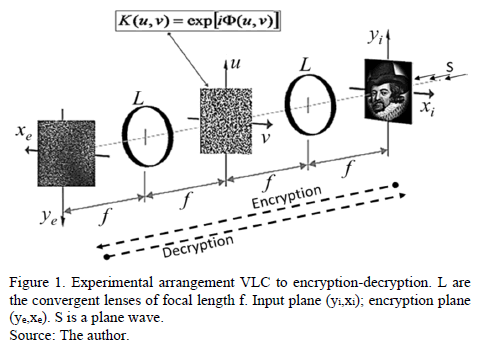Encryption techniques should have two equally important features: 1) resistance to attacks on the encrypted information, and 2) they should allow recipient to decrypt the information without difficulty. In previous works , we implemented this type of encryption arrangement using the encryption-keys in rectangular coordinates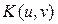. Then, we observed that the decryption is a variant operation with the rotation of the key. In practice, this variance is a problem that needs to be solved. I propose a solution to the problem using a decomposition of the key in circular harmonics.

Fig. 2 shows a result of variance of the decryption operation with the rotation of a key in rectangular coordinates. A rotational variance encryption processor, due to rotation of the key may be measured in terms of the IOR parameter given by eq.(1):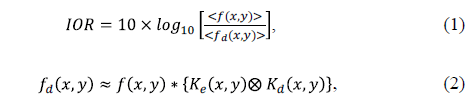Where,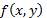is the input image and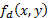is the decrypted image,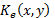is the encryption key, and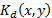is the decryption key. * is the convolution operator and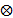is the correlation operator. If IOR = 0 dB, meaning that the output image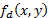is approximately equal to the input image. Analyzing eq.(2), the system will decrypt only if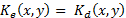, then the operation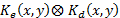generates an autocorrelation peak that does not distort the image. But if we rotate the key, then the result of thecorrelation will be a noise, which is convolved with the image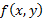, and therefore the result is a distorted image. In this work, it was determined that the level of this distortion depends on the angle of rotation of the decryption key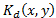. This occurs because the correlation product is variant with rotation functions.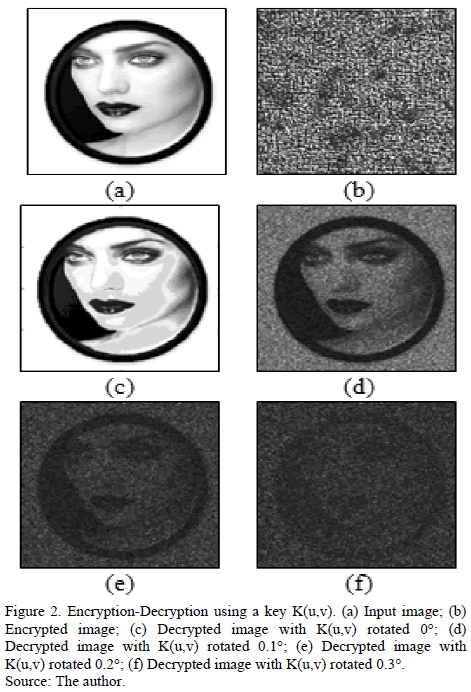The results shown in Fig. 2 correspond to a key in rectangular coordinates, and Fig. 3 is the corresponding calculation of IOR vs the rotation angle of the same key. Note that rotation of 0.2 degrees, corresponds to a value of IOR = 1.25dB, which corresponds to the image in Fig. 2(e), and here it is observed that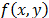is highly distorted. Now, for angles greater than 0.2 degrees, the level of distortion is such that there is no decrypted image, as with the result in Fig. 2(f). The solution is then decomposed into harmonic circular key, and thus the operation of decryption increases tolerance to rotation of the key. Furthermore, we can use IOR as a relative measure of the level of distortion of the decrypted image. Thus, we can see in Fig. 3 that as we increase the angle of rotation of the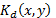key, the value of IOR increases.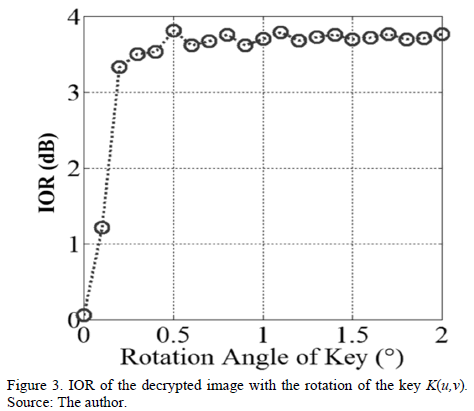2. Encryption with circular harmonic key

An image can be expressed in polar coordinates for circular harmonic components [7,8]. Then we can consider the key in polar coordinates, so the key takes the form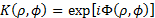, where F must contain a distribution of random values. Thus, the key can be decomposed into harmonic circular, as follows: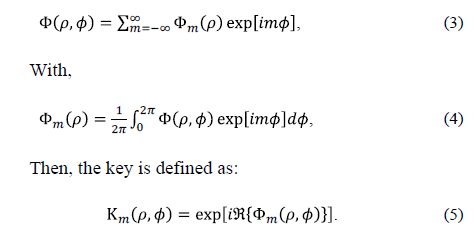Where Â{-} is the real part of Fm. Fig. 4 is an example of decomposition into circular harmonics of m=1 order. Fig. 5 shows the decomposition of m=1 order, of an encryption key given in rectangular coordinates in Fig. 5(a).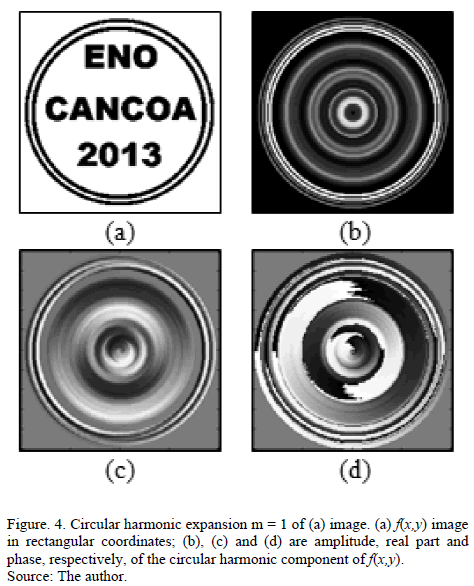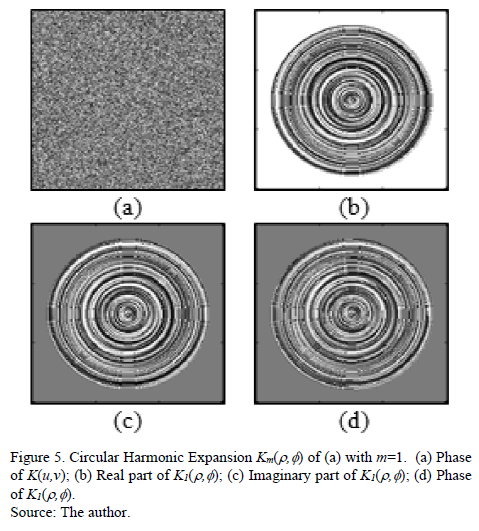2.1. Encryption-Decryption results with circular harmonics key

The block diagram of the cryptography system (Fig. 6) was implemented in Matlab(R2012b). Fig. 7 is a result of encryption-decryption using a key Km(r,f) (Fig. 5). These results indicate that the decryption operation increased the level of tolerance with respect to the case of the key in rectangular coordinates.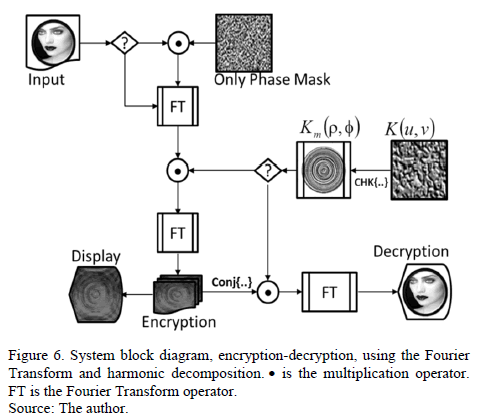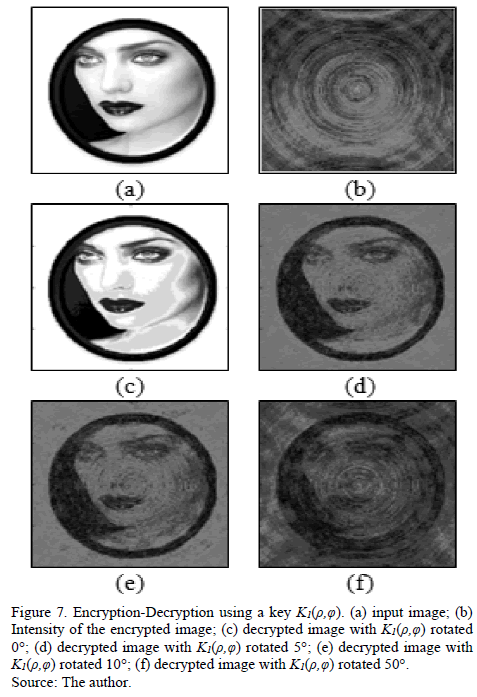Fig. 7(b) shows the intensity of the encrypted image. Note that the intensity of the encrypted image has the appearance of a deterministic image. However, the values are totally random (see Fig. 8). The output of the processor delivers an array of complex values. So the encrypted image is composed of three parts: a real values matrix, an imaginary values matrix and a phase values matrix.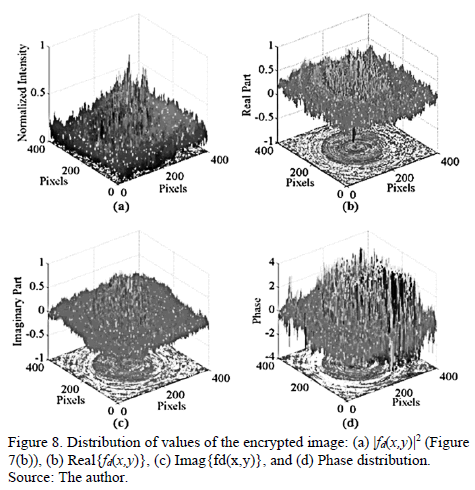Fig. 9 is the relationship IOR between rotation angles of the decryption key. This analysis corresponds to Figs. 7(c)-(f). Note that for an output of IOR = 2dB, a rotation of the key » 41 degrees is necessary. Thus, tolerance decryption operation is much higher with respect to the key in case of rectangular coordinates. So then encryption with keys in polar coordinates is a solution to the problem of variance in the decryption of the optical arrangements, and is also useful for fully digital encryption.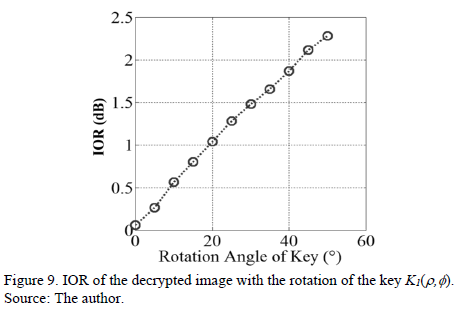The order of the harmonic decomposition can be handled as an additional variable that increases the difficulty of breaking the encryption information.

3. Conclusions

In sum, this study show that the encryption processor based on the Fourier transform is a variant with key rotation. It was demonstrated that a solution to this variance is to expand the key into circular harmonics. The proposed technique increases the tolerance of the processor with the rotation of the key. The key in rectangular coordinates allows a tolerance to rotation of about 0.2 degrees, while with the key in circular harmonics, tolerance increases to about 40 degrees. Thus, the decomposition of the key circular harmonics solves the particular problem of optical encryption processor based on the phenomenon of diffraction, because the decryption key must be physically positioned with high accuracy, as can be concluded from the simulation results presented in this report.

Furthermore, the proposed technique can also be used in digital encryption algorithms, considering that the encrypted image is not deterministic, as it appears to be if only the appearance of the encrypted image is observe. If we analyze the distribution of values of the amplitude and phase of the encrypted image, we find that these are really random. Failing that, the quality of the image encrypted with the key in circular harmonics can be an element of distraction to break the code attacks.

References

 Vanderlugt, A., Signal detection by complex spatial filter, IEEE IT-10, pp.139-146, 1964.         [ Links ]

 Refregier, P. and Javidi, B., Optical image encryption based on input plane and Fourier plane random encoding, Opt. Lett. 20 (7), pp.767-769, 1995. http://dx.doi.org/10.1364/OL.20.000767        [ Links ]

 Matoba, O. and Javidi, B., Encrypted optical storage with angular multiplexing, Appl. Opt. 38 (35), pp.7288-7293, 1999. http://dx.doi.org/10.1364/AO.38.007288.         [ Links ]

 Lin, C., Shen, X., Tang, R. and Zou, X., Multiple images encryption based on Fourier transform hologram, Opt. Commun. 285 (6), pp.1023-1028, 2012. DOI: 10.1016/j.optcom.2011.10.046        [ Links ]

 Tebaldi, M., Furlan W.D., Torroba, R. and Bolognini, N., Optical-data storage-readout technique based on fractal encrypting masks, Opt. Lett. 34 (3), pp. 316-318, 2009. http://dx.doi.org/10.1364/OL.34.000316        [ Links ]

 Rueda, J.E. and Romero, A.L., Optical cryptography using Fresnel diffraction and phase conjugation, Revista DYNA. Facultad de Minas, Universidad Nacional de Colombia, Sede Medellín, Colombia, 80 (181), pp. 25-30, 2013.         [ Links ]

 Hsu, Y.N., Arsenault, H.H. and April, G., Rotation-invariant digital patter recognition using circular harmonic expansion, Appl. Opt. 21(22), pp. 4012-4015, 1982. http://dx.doi.org/10.1364/AO.21.004012        [ Links ]

 Gualdron O. and Arsenault H.H., Phase dirived circular harmonic filter, Opt. Commun. 104(1-3), pp.32-34, 1993. DOI: 10.1016/0030-4018(93)90100-J        [ Links ]

 Hennelly, B. and Sheridan, J.T. Optical image encryption by random shifting in fractional Fourier domains. Optics Letters 28(4), pp.269-271, 2003. http://dx.doi.org/10.1364/OL.28.000269         [ Links ]

 Salazar, A., Rueda, J.E. and Lasprilla, M., Encriptación por conjugación de fase en un BSO utilizando señales ópticas de baja potencia, Revista Colombiana de Física 34 (2), pp.636-640, 2002.         [ Links ]

J.E. Rueda-Parada, completed his BSc in Physics in 1993, an MSc Physics in 1996, and a PhD Physics in 2002, all of them from the Universidad Industrial de Santander, Colombia. He finished his postdoctoral studies in February 2015, at the Institute of Physics of São Carlos, University of São Paulo, Brazil. Currently, he is a full Professor at the Physics Department, Faculty of Basic Sciences, Universidad de Pamplona, Colombia. His research interests include: growth of mono-crystalline fibers, photorefractive optics, holography, wave mixing, and optical encryption and digital image processing.